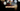# Leetcode | Solution of Contains Duplicate II in JavaScript

March 31st, 2020
|

In this post, we will solve the problem contains duplicate II from leetcode and compute it's time and space complexities. Let's begin.

# Problem Statement

The question can be found at leetcode contains duplicate II problem.

The problem states that we are given an array of numbers and we need to find if there are any two indices whose values are same and their absolute difference is at most K

# Constraints and challenges

• The difference in indices is at most K
• There are a couple of edge cases

# Solution

Initially, the question looks very straight forward.

• Keep iterating over the array and pushing values and indices in a map
• once we encounter a value which is already in the map, we check for the difference in indices.

This approach works well if there's a duplicate in the array but is limited to cases where a value is present at most twice in the array

Let's take an example

``[1,0,1,1]``

In the array taken above, we loop through the array and keep `1,0` in the map with index `0,1` respectively. When we reach at second `1`, the absolute difference between both indices of 1s is`|2-0| = 2`, now if the value of `k` was `1`, this condition would fail. However, we have another `1` after that and the absolute difference would be `3` if we compare it to the first one. But, if we compare it to the second `1`, the absolute difference would be `|2-3|=1`, which would satisfy the condition.

To tackle this situation, we will have to place another check i.e. no matter what the case is(recurring value or not) we will update the value and index in the map. That will ensure we keep on updating the values in the map and the absolute difference will be as small as possible.

Phew!!! It was a tricky one, I hope I was able to explain it clearly. Please check the video below in case you still have doubts.

We have discussed the approach, I urge you to go ahead on leetcode and give it another try.If you are here, it means something went wrong in implementation or you are just too lazy. In any case, let's see a simple implementation of the above logic.
``````var containsNearbyDuplicate = function (nums, k) {
const map = new Map();

for (let i = 0; i < nums.length; i++) {
if (map.has(nums[i])) {
const j = map.get(nums[i]);

if (Math.abs(i - j) <= k) {
return true;
}
}

map.set(nums[i], i);
}

return false;
};``````

Let's look at the solution.

First, we create a map. Next, we loop over all the elements in the array. If the value exists in the map, we get its index and find the absolute difference with current index. If it satisfies the condition given in the question, we return true and program exits.

In case the condition fails, or the number is not present in the map, we update or insert the index in the map(to handle the edge case where a number is present more than twice in the array).

If we jump out of the loop, that means the condition was never met. So we return false and exit.

On submission, here are the stats

``````Status: Accepted
Runtime: 64ms
Memory: 40MB``````

## Time and space complexity

### Time complexity

We are using a simple for loop to iterate over the array and look up in a map is constant time. So, the time complexity is O(n).

### Space complexity

We are using extra space to keep the elements of the array in the map, so space complexity is O(n).

# Summary

So, we solved the contains duplicate II using a simple loop and a map and finally, calculated the time and space complexities.

I hope you enjoyed solving this question. This is it for this one, complete source code for this post can be found on my Github Repo. Will see you in the next one.